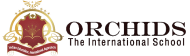Enquire Now

# Marks Percentage Calculator

A marks percentage calculator is a tool for calculating the percentage of marks obtained in a test. It is useful for students to keep track of their overall percentage scores.
The Percentage is:
How to Use a Marks Percentage Calculator?
1. Enter the marks you have scored in the examination.
2. Enter the Total marks.
3. Press Calculate to get your result.
4. You must click the reset button to enter the new information.
Benefits of Using Marks Percentage Calculator
1. It is simple to get the result without plotting any formulas.
2. It is possible to manage large calculations and obtain accurate results.
3. It helps you save time and do numerous calculations in less time.
How to Calculate Percentage of Marks?
A fraction of 100 is used to express a number as a percentage. We divide the total scores by the marks obtained on the test to determine the percentage of the marks, and we multiply the result by 100.
Percentage Formula
Percentage = (Scored marks/ Total marks) x 100
Example:
Problem:
A student scored 395 marks out of 500. Find the percentage.
Solution:
Percentage = (Scored marks/Total marks) x 100 = (395/500) x 100 = 79%

## FAQs

##### What Number is 60% of 500?

| K12 Techno Services ®

ORCHIDS - The International School | Terms | Privacy Policy | Cancellation